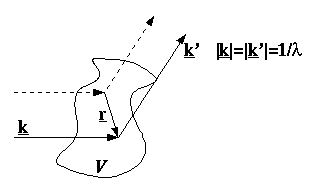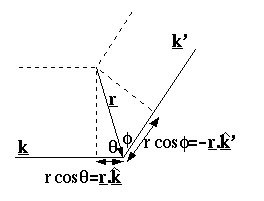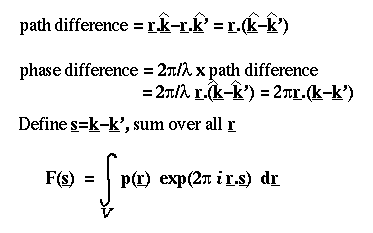## Scattering Theory

What is the connection between X-ray diffraction and Fourier transforms? To answer this question we must deduce how an arbitrary solid will scatter an incident wave.

Consider an arbitrary solid. A plane wave is incident upon this solid, and is scattered from every point in the solid. We will consider the diffracted wave along a particular direction, and calculate the total scattering by summing the scattering along that direction from each point in the solid.

Let the incident beam direction be represented by a vector k. The diffracted beam along the chosen direction will be k'. For convenience, we set the magnitude of these vectors to the reciprocal of the wavelength of the beam.Now we must sum the scattering from the whole solid. First, we pick an origin within the solid. Then, we calculate the difference in path length between a beam scattered from an arbitrary point in the solid and an imaginary beam scattered from the origin.This difference in path length is translated into a phase difference between the two waves. We can then sum the scattering over the whole solid, taking into account the strength of scattering from each point, p(r), and the complex representation of the phase shift. If we substitute a new vector s for the difference between k and k', then we obtain a standard Fourier integral:Back to the index.